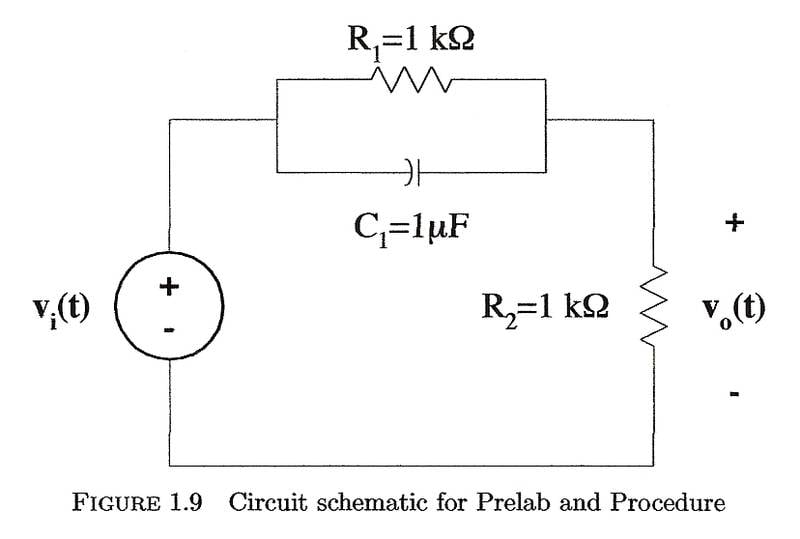# Circuit Analysis: Deriving Transient Expressions

• Engineering
• amiv4

## Homework Statementanalytically derive the transient expressions for the following
1. the output voltage vot for t > 0
2. the power absorbed by each resistor and capacitor

## The Attempt at a Solution

I'm not sure where to start. Don't i need to know what vi(t) is?

I am new to circuit anaylsis too, but I am pretty sure you do, perhaps someone with more experience might advised other way.
Was no equation given being that both voltages are functions of time, t?

The only thing I can think of is that voltage can also be found by

$V = IR$
V(Voltage) = I(Current) x R(Resistance)

## Homework Statementanalytically derive the transient expressions for the following
1. the output voltage vot for t > 0
2. the power absorbed by each resistor and capacitor

## The Attempt at a Solution

I'm not sure where to start. Don't i need to know what vi(t) is?

Write the KCL equation for that upper right node -- it will give you a differential equation to solve.